Olympiad Test: Geometrical Shapes And Angles - 1

# Olympiad Test: Geometrical Shapes And Angles - 1 - Class 5

Test Description

## 20 Questions MCQ Test National Cyber Olympiad Class 5 - Olympiad Test: Geometrical Shapes And Angles - 1

Olympiad Test: Geometrical Shapes And Angles - 1 for Class 5 2023 is part of National Cyber Olympiad Class 5 preparation. The Olympiad Test: Geometrical Shapes And Angles - 1 questions and answers have been prepared according to the Class 5 exam syllabus.The Olympiad Test: Geometrical Shapes And Angles - 1 MCQs are made for Class 5 2023 Exam. Find important definitions, questions, notes, meanings, examples, exercises, MCQs and online tests for Olympiad Test: Geometrical Shapes And Angles - 1 below.
 1 Crore+ students have signed up on EduRev. Have you?
Olympiad Test: Geometrical Shapes And Angles - 1 - Question 1

### The angle 89° is:

Detailed Solution for Olympiad Test: Geometrical Shapes And Angles - 1 - Question 1

Since 89° is less than 90°, it is acute.

Olympiad Test: Geometrical Shapes And Angles - 1 - Question 2

### The angle 234° is:

Detailed Solution for Olympiad Test: Geometrical Shapes And Angles - 1 - Question 2

Since 234° is greater than 180°, it is reflex.

Olympiad Test: Geometrical Shapes And Angles - 1 - Question 3

### The angle 98° is:

Detailed Solution for Olympiad Test: Geometrical Shapes And Angles - 1 - Question 3

Since 98° is greater than 90° but less than 180°, it is obtuse

Olympiad Test: Geometrical Shapes And Angles - 1 - Question 4

Which is closest to the size of angle AOB?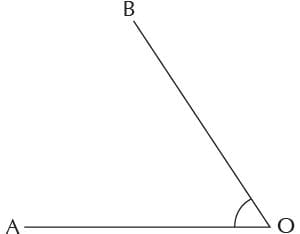Detailed Solution for Olympiad Test: Geometrical Shapes And Angles - 1 - Question 4

Measuring from O (Alphabet) in the direction of the arrow, angle AOB = 57°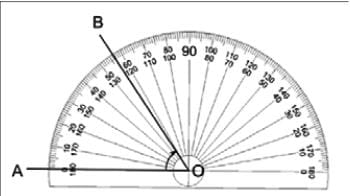Olympiad Test: Geometrical Shapes And Angles - 1 - Question 5

Which is closest to the size of angle COD?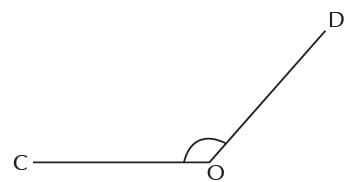Detailed Solution for Olympiad Test: Geometrical Shapes And Angles - 1 - Question 5

Starting from O, and measuring in the direction of the arrow, angle COD = 131°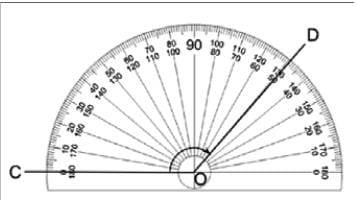Olympiad Test: Geometrical Shapes And Angles - 1 - Question 6

Which is closest to the size of reflex angle FOE?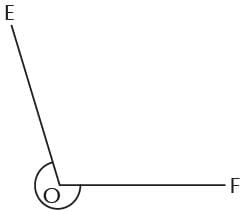Detailed Solution for Olympiad Test: Geometrical Shapes And Angles - 1 - Question 6

Reflex Angle FOE can be split into two: Angle FOE = Angle FOG + Angle GOE
Angle FOG is a straight angle
∴ ∠FOG= 180°. Now, starting from O and measuring in the direction of the arrow
Angle GOE = 74°
So Reflex Angle FOE = Angle FOG + Angle GOE = 180° + 74° =254°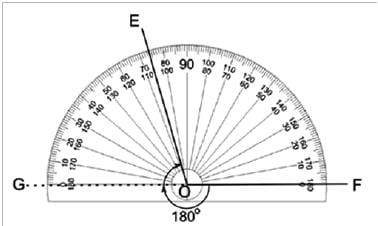Olympiad Test: Geometrical Shapes And Angles - 1 - Question 7

For the angle shown in the diagram, the arrow points to its: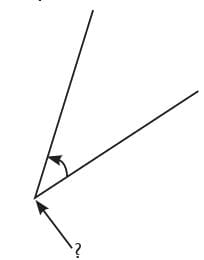Detailed Solution for Olympiad Test: Geometrical Shapes And Angles - 1 - Question 7

The vertex (plural vertices) is a corner point of two rays.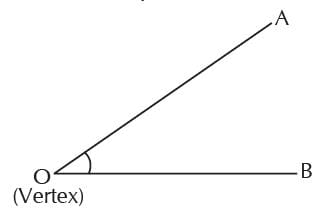Olympiad Test: Geometrical Shapes And Angles - 1 - Question 8

By using the three letters on the shape that define the angle, angle α is written as: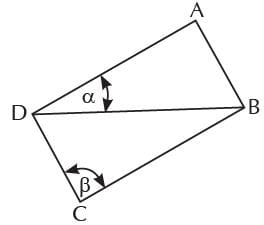Detailed Solution for Olympiad Test: Geometrical Shapes And Angles - 1 - Question 8

The middle letter is where the angle actually is (its vertex), which is D. Therefore we could write the angle as ∠BDA or ∠ADB.

Olympiad Test: Geometrical Shapes And Angles - 1 - Question 9

By using the three letters on the shape that define the angle, angle β is written as: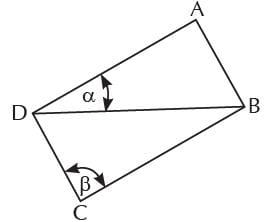Detailed Solution for Olympiad Test: Geometrical Shapes And Angles - 1 - Question 9

The middle letter is where the angle actually is (its vertex), which is C. Therefore we could write the angle as ∠BCD or ∠DCB.

Olympiad Test: Geometrical Shapes And Angles - 1 - Question 10

If two acute angles are added together, which of the following is NOT possible for their sum:

Detailed Solution for Olympiad Test: Geometrical Shapes And Angles - 1 - Question 10

An acute angle is less than 90°.
If you add two acute angles then
(i) The sum could be acute, example: 10° + 20° = 30° (an acute angle).
(ii) The sum could be right, example: 40° + 50° = 90° (a right angle).
(iii) The sum could be obtuse, example: 30° + 80° = 110° (an obtuse angle).
(iv) the sum could NOT be straight because each acute angle is less than 90° and a straight angle is 180°, example: 89° + 89° = 178° which is less than a straight angle.

Olympiad Test: Geometrical Shapes And Angles - 1 - Question 11

Which one of the following angles is not acute?

Detailed Solution for Olympiad Test: Geometrical Shapes And Angles - 1 - Question 11

An acute angle is less than 90°. 91° is more than 90°, so it is not acute.

Olympiad Test: Geometrical Shapes And Angles - 1 - Question 12

Which one of the following angles is acute?

Detailed Solution for Olympiad Test: Geometrical Shapes And Angles - 1 - Question 12

An acute angle is less than 90° i.e. it is less than a right angle. Half a right angle (45°) is less than a right angle, so it is acute.

Olympiad Test: Geometrical Shapes And Angles - 1 - Question 13

How many acute angles are there in the diagram?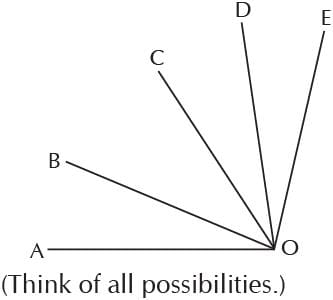Detailed Solution for Olympiad Test: Geometrical Shapes And Angles - 1 - Question 13

The following angles are all less than 90°, so are acute:     ∠AOB, ∠BOC, ∠COD,  ∠DOE,
∠AOC, ∠AOD, ∠BOD, ∠BOE and ∠COE
Therefore, there are 9 acute angles altogether. Note that ∠AOE is obtuse.

Olympiad Test: Geometrical Shapes And Angles - 1 - Question 14

How many acute angles are there in this pentagram?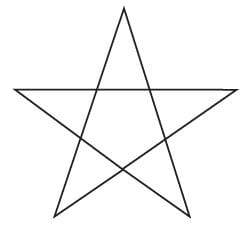Detailed Solution for Olympiad Test: Geometrical Shapes And Angles - 1 - Question 14

Each of the five triangles at the points of the pentagram has three acute angles. So there are 5 × 3 = 15 acute angles altogether in the pentagram. (All other angles are not acute)

Olympiad Test: Geometrical Shapes And Angles - 1 - Question 15

How many acute angles are there in the diagram?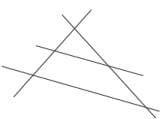Detailed Solution for Olympiad Test: Geometrical Shapes And Angles - 1 - Question 15

The marked angles are all less than 90°, so are acute: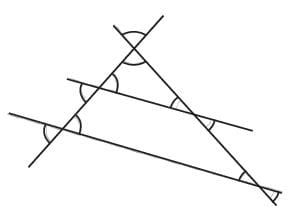Therefore, there are 10 acute angles altogether.

Olympiad Test: Geometrical Shapes And Angles - 1 - Question 16

How many acute angles are there in the diagram?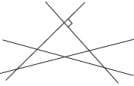Detailed Solution for Olympiad Test: Geometrical Shapes And Angles - 1 - Question 16

The angles marked with arcs are all less than 90°, so are acute. The four angles around the top point are all right angles. So there are 10 acute angles altogether.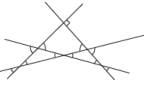Olympiad Test: Geometrical Shapes And Angles - 1 - Question 17

How many right angles are there in the diagram?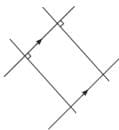Detailed Solution for Olympiad Test: Geometrical Shapes And Angles - 1 - Question 17

Because one pair of lines is parallel (that is what the two arrows mean) and the other two lines are perpendicular to them (the little boxes mean right angles), the shape inside the four lines must be a rectangle. And there are 4 right angles at each of the four points where lines intersect. So there are 4 × 4 = 16 right angles altogether.

Olympiad Test: Geometrical Shapes And Angles - 1 - Question 18

How many right angles make two full rotations?

Detailed Solution for Olympiad Test: Geometrical Shapes And Angles - 1 - Question 18

A full rotation consists of four right angles. So two full rotations consist of eight right angles.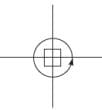Olympiad Test: Geometrical Shapes And Angles - 1 - Question 19

Which one of the following angles is not obtuse?

Detailed Solution for Olympiad Test: Geometrical Shapes And Angles - 1 - Question 19

An obtuse angle is more than 90° but less than 180°.182° is more than 180°, so it is not obtuse.

Olympiad Test: Geometrical Shapes And Angles - 1 - Question 20

How many obtuse angles are there in the diagram?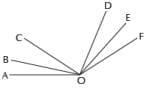Detailed Solution for Olympiad Test: Geometrical Shapes And Angles - 1 - Question 20

The following angles are obtuse: ∠AOD, ∠AOE, ∠AOF, ∠BOD, ∠BOE,
∠BOF, ∠COE and ∠COF. Therefore, there are 8 obtuse angles altogether.

## National Cyber Olympiad Class 5

1 videos|22 tests